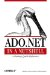There are three methods that can add new rows to the DataTable . The NewRow( ) method creates a new empty DataRow with the same schema as the DataTable . After creating the row, it can be added to the DataTable using the Add( ) method of the DataRowCollection : ` // create the target table DataTable dt = new DataTable("MyTable"); dt.Columns.Add("Column1", typeof(System.Int32)); dt.Columns.Add("Column2", typeof(System.String)); // create and add a new row to the table DataRow newrow = dt.NewRow(); newrow["Column1"] = 1; newrow["Column2"] = "Row 1"; dt.Rows.Add(newrow); ` The LoadDataRow( ) method takes an array of values and attempts to find a row with a matching primary key. If the primary key is found, the values replace the existing data for the row; otherwise a new row is added. The LoadDataRow( ) method takes a Boolean AcceptChanges argument. If the AcceptChanges value is true , AcceptChanges is called to accept all changes for both inserted and modified rows. If AcceptChanges is false , the DataRowState fields of newly added rows are marked as insertions, while changes to existing rows are marked as modifications. The BeginLoadData( ) method turns off all constraints, notifications, and index maintenance for the DataTable while data is loaded; the EndLoadData( ) method turns them back on. Calling BeginLoadData( ) and EndLoadData( ) methods might result in performance improvements when adding a series of DataRows to the DataTable using the LoadDataRow( ) method. If there are constraint violations when the EndLoadData( ) method is called, a ConstraintException is raised. The following example illustrates these methods: ` // create the target table DataTable dt = new DataTable("MyTable"); dt.Columns.Add("Column1", typeof(System.Int32)); dt.Columns.Add("Column2", typeof(System.String)); // add two rows to the DataTable dt dt.BeginLoadData(); dt.LoadDataRow(new Object[]{1,"Row 1"}, false); dt.LoadDataRow(new Object[]{2,"Row 2"}, false); dt.EndLoadData(); ` Finally, the ImportRow( ) method accepts a DataRow object argument and either adds the row to the table or updates an existing row with a matching primary key in the table, using the existing schema and preserving the existing DataRowState of the row: ` // create the target table DataTable dt = new DataTable("MyTable"); dt.Columns.Add("Column1", typeof(System.Int32)); dt.Columns.Add("Column2", typeof(System.String)); DataRow newrow = dt.NewRow(); newrow["Column1"] = 1; newrow["Column2"] = "Row 1"; dt.Rows.ImportRow(newrow); `